Courses

# Test: Second Order RLC Circuits- 2

## 15 Questions MCQ Test Topicwise Question Bank for Electrical Engineering | Test: Second Order RLC Circuits- 2

Description
This mock test of Test: Second Order RLC Circuits- 2 for Electrical Engineering (EE) helps you for every Electrical Engineering (EE) entrance exam. This contains 15 Multiple Choice Questions for Electrical Engineering (EE) Test: Second Order RLC Circuits- 2 (mcq) to study with solutions a complete question bank. The solved questions answers in this Test: Second Order RLC Circuits- 2 quiz give you a good mix of easy questions and tough questions. Electrical Engineering (EE) students definitely take this Test: Second Order RLC Circuits- 2 exercise for a better result in the exam. You can find other Test: Second Order RLC Circuits- 2 extra questions, long questions & short questions for Electrical Engineering (EE) on EduRev as well by searching above.
QUESTION: 1

### If the value of current i(t) for the circuit shown below is i(t) -20 e-2t, then the voltage source v(t) will be given by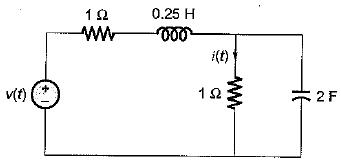Solution: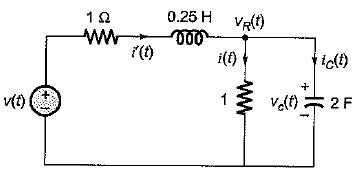vR(t) = i(t) x 1 = -20 e-2t = vc(t)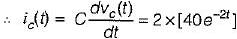= 80 e -2t
Now, i'(t) = i(t) + ic(t)
= -20 e-2t + 80 e-2t = 60 e-2t
So,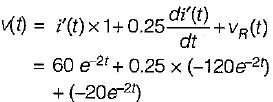or,
v(t) = (60 - 30 - 20) e-2t = 10 e-2t

QUESTION: 2

### The circuit shown in figure is critically damped.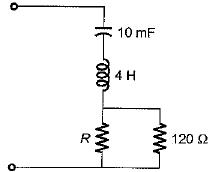The value of R is

Solution: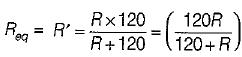For an RLC series circuit to be critically damped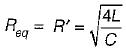or,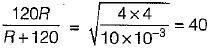or, 120 R = 40 ( R + 120)
or, 3 R = R + 120 or R = 60 Ω

QUESTION: 3

### The voltage across a passive element in an electric circuit is given by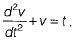It is given that V(0+)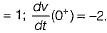The value of v(t) is

Solution:

Given,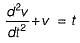Taking Laplace transform on both sides,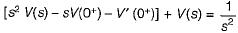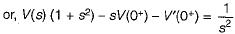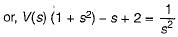or,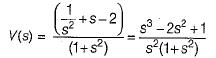or,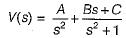Using partial fraction expansion,
A = 1, B = 1,
C = - 3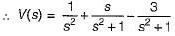So, V(t) = (t + cost - 3 sint)

QUESTION: 4

A voltage is given by V(s) = 1/S(S + a). If V(∞) = 2 volts, then V(1) is

Solution:

Given,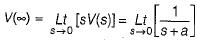= 1/a = 2
or, a = 0.5
Thus,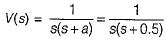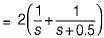or v(t) = 2(1 - e - 0.5t)
Hence, v(1) = 2(1 - e-0.5)

QUESTION: 5

Pole of a network is frequency at which

Solution:
QUESTION: 6

Transient current in an R-L-C circuit oscillatory when

Solution:

Characteristic equation of series RLC circuit is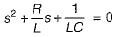Here,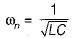and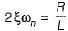or,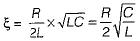For oscillatory response,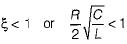or,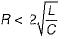QUESTION: 7

Which of the following conditions are necessary for the validity of initial value theorem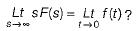Solution:
QUESTION: 8

The poles and zeros of the transfer function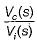for the circuit shown below are located a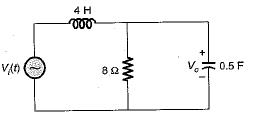Solution:

Applying KCL at the given node in s-domain, we have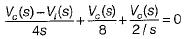or,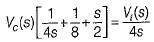or,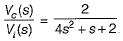Hence, there is no-zero and poles are at 4 s2 + s + 2 = 0
or,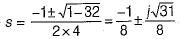QUESTION: 9

Assertion (A): Laplace transformation finds its application in solving the transient behaviour of the electric circuits.
Reason (R): The transient response of an electrical circuit can best be described by a linear differential equation.

Solution:

Because the transient response of an electrical circuit can best be described by a differential equation hence, Laplace transformation finds its application in solving the transient behaviour of the electric circuits.

QUESTION: 10

The final value of f(t) = e-t (sin 2t + cos 5t) is

Solution:

f(t) = e-t sin 2t + e-2t cos 5t
∴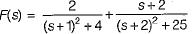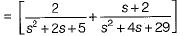∴ Find value of f(t) is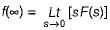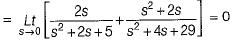QUESTION: 11

At t = 0+ with zero initial condition, the voltage across 20 Ω resistor is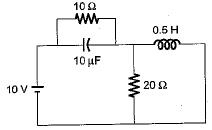Solution:

At t = 0+, capacitor will act as short circuit while inductor an open circuit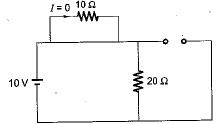∴ V20Ω = 10 volt

QUESTION: 12

Assuming zero-initial condition vc(t) in the given circuit will be given by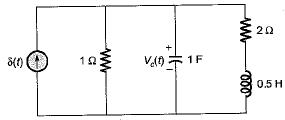Solution:

Applying node analysis,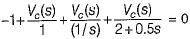or,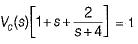or,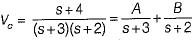Here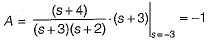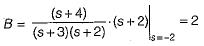∴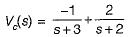or,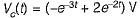QUESTION: 13

The initial and final value of current through the resistor Rina series RLC circuit with unit step input and zero initial condition are respectively

Solution: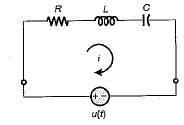At t = 0, inductor act as open circuit i(0+) = 0 A
At t = capacitor act as open circuit i(∞) = 0 A

QUESTION: 14

The value of current through R at t = ∞ is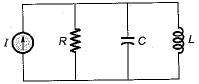Solution:

At t = ∞, inductor will act as short circuit anc capacitor open circuit.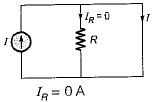QUESTION: 15

Consider the following statements for a series RLC circuit excited with a voltage v(t)
1. For ξ, = 0, there is no-loss in the circuit.
2. The damping ratio of the circuit is independent of resistance R of the circuit.
3. The response of the circuit is oscillator if ξ value is more than unity.

Q. Which of the above statements is/are true?

Solution: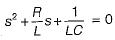(characteristic equation)
Therefore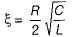If R = 0, ξ = 0 (vice-versa) and for ξ < 1, response will be oscillatory.